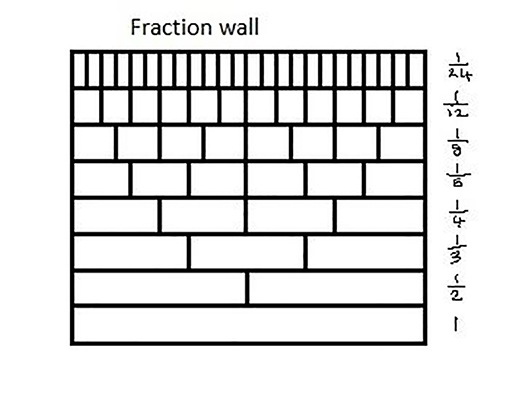Teaching mathematics

Start this free course now. Just create an account and sign in. Enrol and complete the course for a free statement of participation or digital badge if available.

Free course

# The fraction wall

Figure 1 shows a fraction wall divided into strips of the same length which can be split into equal parts. The fraction wall can be used to identify equivalent fractions.Figure 1 Fraction wall

## Activity 2 Finding equivalent fractions

Timing: Allow 5 minutes

Use the fraction wall to find as many fractions as you can that are equivalent to .

Use the fraction wall to find as many fractions as you can that are equivalent to .

TM_1